8 Comparisons of MorphologyFor each comparison, the left image is an atomic shape representing one of eight different excitation states. The images to the right are photographs and schematic models of planetary nebula star systems.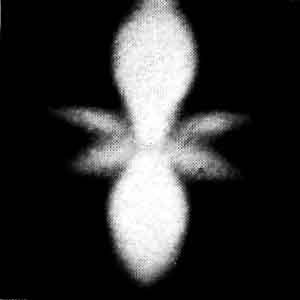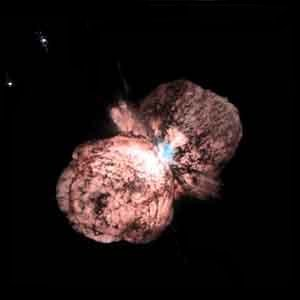n=4, l=4, m=0Eta Carina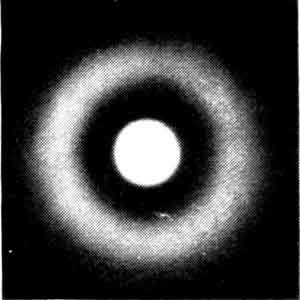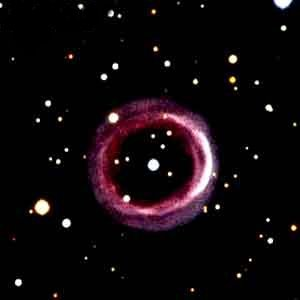n=2, l=0, m=0SHAP-1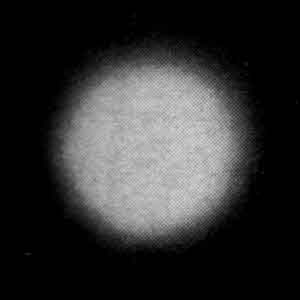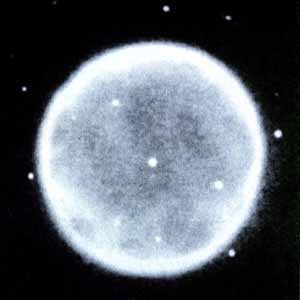n=1, l=m=0A-39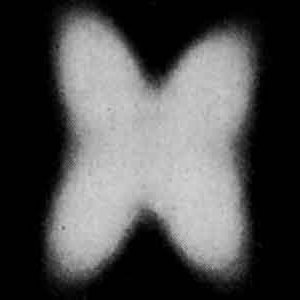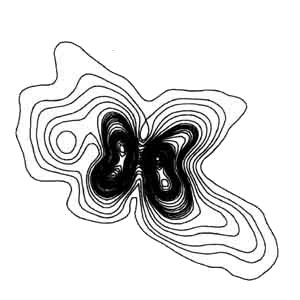n=3, l=3, m=1IRAS 04302+2247
(schematic)n=2, l=2, m=0NGC6563NGC6058n=l=m=2M1-92NGC6826(schematic)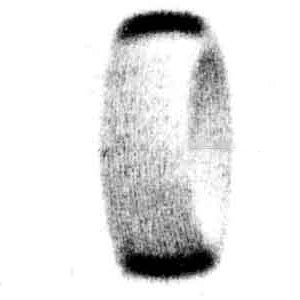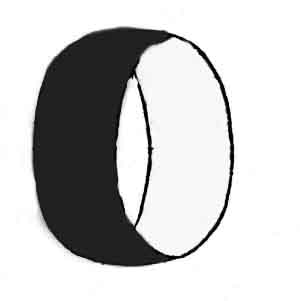n=3, l=2, m=+/-2NGC6772
(schematic)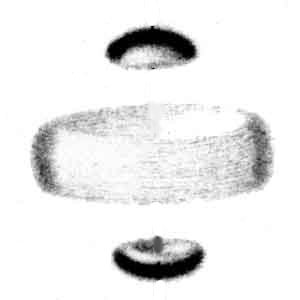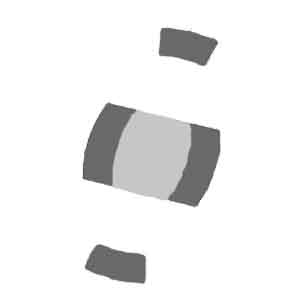n=3, l=2, m=0NGC7026
(schematic)It should be emphasized that these are not uncommon examples of atomic or planetary nebula morphologies. To the contrary, they are THE MOST COMMON SHAPES for both types of system: spheriod, bipolar "propeller", caps, "butterfly", toroid, sphere-within-a-sphere, etc.

It is not necessary to choose rare examples in order to achieve isomorphism. These are archetypal shapes for the atomic and stellar scale systems.

A. ATOMIC MORPHOLOGY1. The far left atomic scale shapes are visual depictions of electron probability densities for the simplest atom, hydrogen, in its lowest energy state and a few excited states. Usually these probability densities are interpreted as abstract probabilities for finding a point-like electron at a given place. Another interpretation (one not favored by most physicists, though Schrodinger himself favored it) is as follows. When electrons become bound to an atom, their mass is spread out into these shapes. What are called abstract probability density distributions are really distributions of the physical mass of the electron for a given energy state.

2. Pictoral examples of these density distributions can be found in the following sources:(a.) H.E. White, Physical Review, Vol. 37, 1931 (p. 1419 and p. 1423). [These images are the widely acknowledged classics]
(b.)R. Eisberg and R. Resnick, Quantum Physics of Atoms, Molecules, Solids, Nuclei, and Particles (2nd Ed.), John Wiley, New York, 1985 (p.251). This Material is used by permission of John Wiley & Sons, Inc. Copyright (R) 1985, John Wiley & Sons, Inc.

B. PLANETARY NEBULA MORPHOLOGY1. It should be kept in mind that planetary nebulae are created when their central stars somewhat violently eject their outer shells. Some loss of the pre-existing symmetry and detailed morphology can be expected for these expanding structures.

2. Excellent collections of planetary nebula images can be found on the internet at www.blackskies.com/links.

3. For a basic discussion of what planetary nebula systems are, see www.astro.washington.edu/balick/WFPC2.

4. As mentioned above, the origin and maintenance of planetary nebula morphologies are still considered to be unsolved problems by professional astrophysicists.

5. The planetary nebula images shown above are recreated from images found on the web, or published in books and journals.